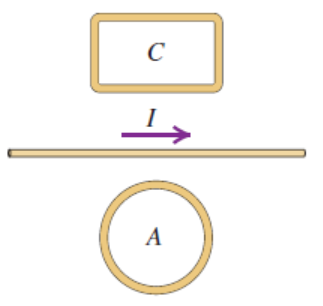# Problem: Two closed loops A and C are close to a long wire carrying a current(a) Find the direction (clockwise or counterclockwise) of the current induced in each loop if/is steadily decreasing.(b) While/ is decreasing, what is the direction of the net force that the wire exerts on each loop? Explain how you obtain your answer

###### FREE Expert Solution

(a)

We apply the right-hand rule. The thumb points in the direction of the current, other fingers in the direction of magnetic field.

To do this, grip coil A with the right hand in a manner that the thumb points in the counterclockwise direction.###### Problem Details

Two closed loops A and C are close to a long wire carrying a current

(a) Find the direction (clockwise or counterclockwise) of the current induced in each loop if/is steadily decreasing.

(b) While/ is decreasing, what is the direction of the net force that the wire exerts on each loop?